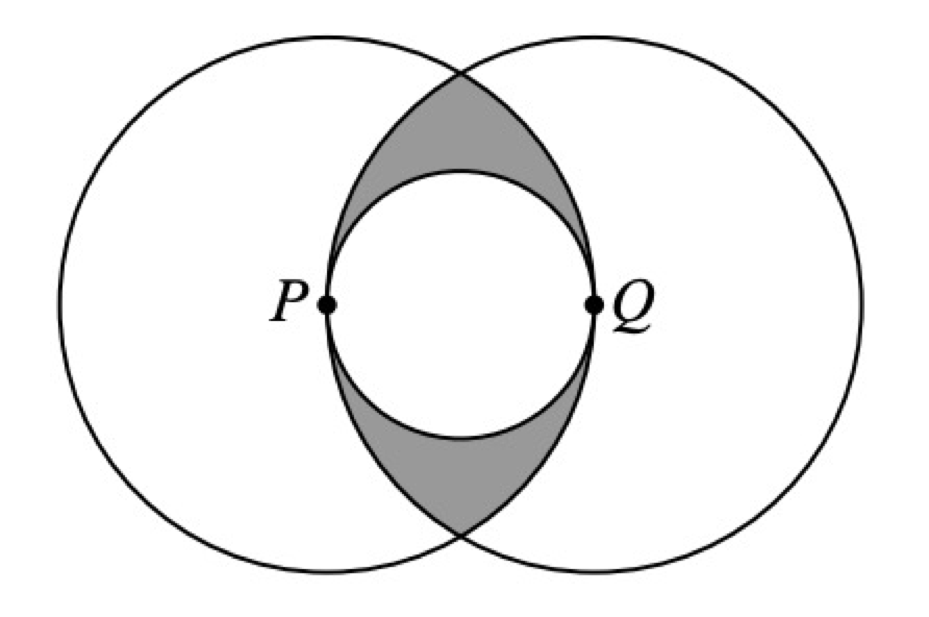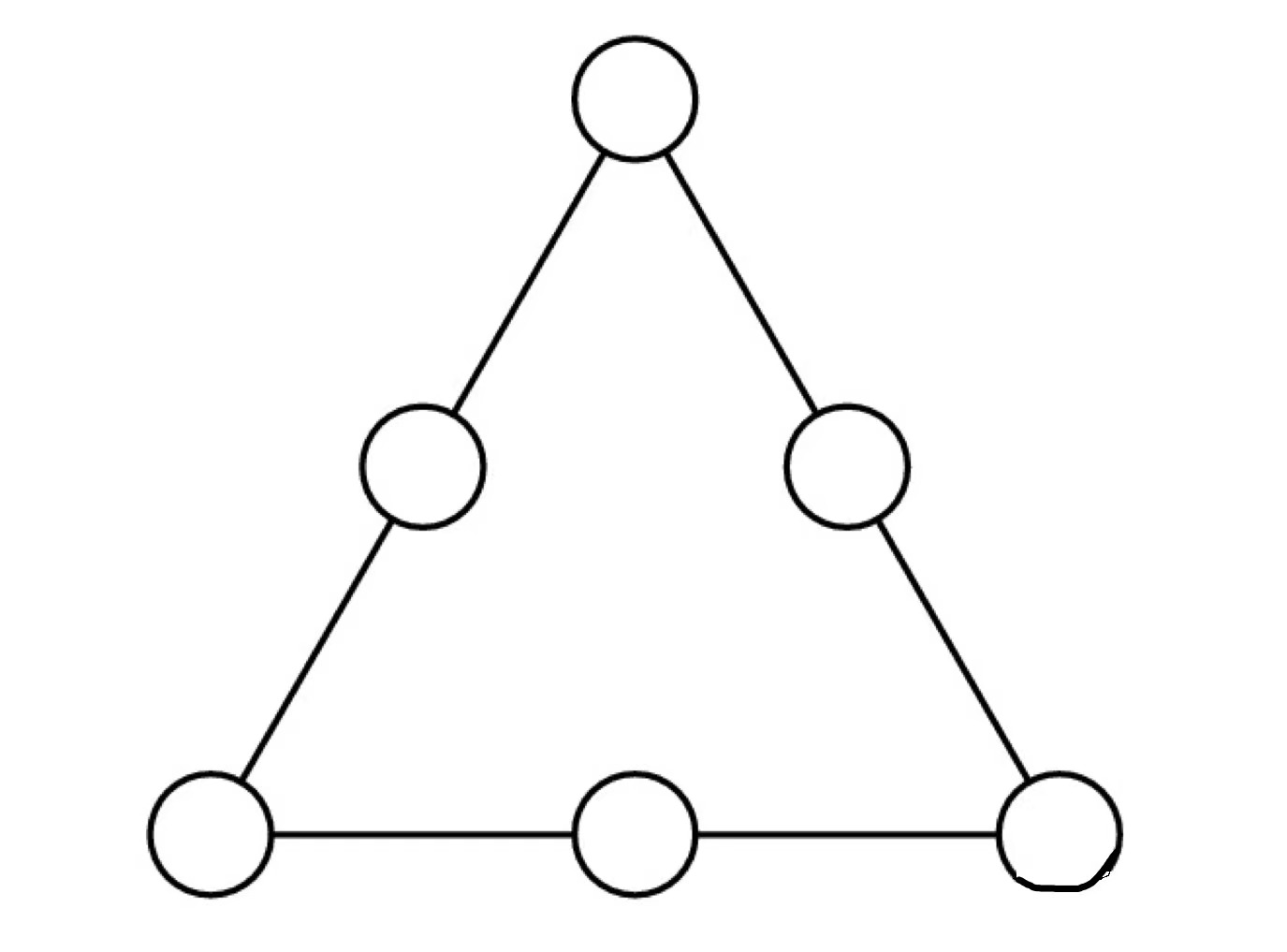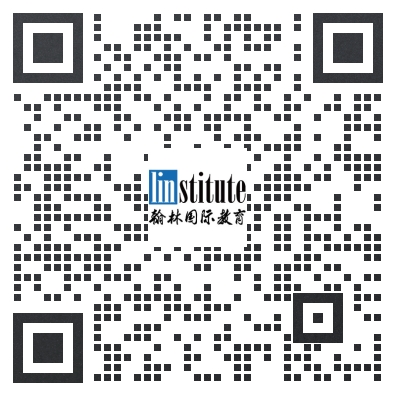# 加拿大滑铁卢数学竞赛真题分享及答案！

1.The digits from 1 to 9 are written in order so that the digit n is written n times. This forms the block of digits 1223334444 · · · 999999999. The block is written 100 times. What is the 1953rd digit written? (2018 Gauss 7)

2.In the diagram, two larger circles with radius 1 have centres P and Q. Also, the smaller circle has diameter PQ. The region inside the two larger circles and outside the smaller circle is shaded. The area of the shaded region is closest to (2018 Pascal 9)3.The number of ways that 135 can be expressed as the sum of at leastt two consecutive positive integers is?

4.In a magic triangle, each of the six whole numbers 10-15 is placed in one of the circles so that the sum, S, of the three numbers on each side of the triangle is the same. The largest possible value for S is5.Two different prime numbers between \$4\$ and \$18\$ are chosen. When their sum is subtracted from their product, which of the following numbers could be obtained?

A)  22

B) 60

C)119

D) 94

E)231

1. 6

2.约等于0.443

3. 7

4. 39

5.C

【扫码联系老师领取报名表】

【免费领取】相关真题及解析，还有不定期的高能讲座等你来参加！